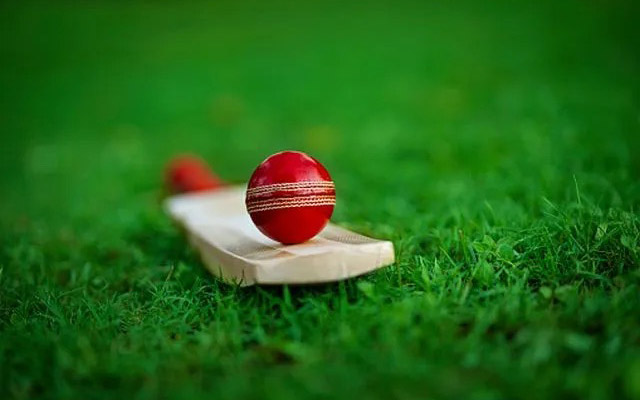# How do you read cricket betting odds?Cricket betting odds play a crucial role in determining the potential payouts and understanding the likelihood of a specific outcome in a cricket match

Cricket betting odds play a crucial role in determining the potential payouts and understanding the likelihood of a specific outcome in a cricket match. If you’re new to cricket betting, it’s essential to learn how to read and interpret these odds effectively. In this article, we will guide you through the process of reading cricket betting odds, enabling you to make informed decisions when placing your bets.

Types of Odds Formats:

Cricket betting odds can be presented in different formats, including decimal, fractional, or moneyline. Understanding each format is essential to grasp the implied probabilities and potential payouts associated with a particular bet.

A. Decimal Odds: Decimal odds represent the total return, including the original stake, for every unit wagered. For example, if a team has odds of 2.50, a winning bet of \$10 would result in a total payout of \$25 (\$10 x 2.50).

B. Fractional Odds: Fractional odds are presented as a fraction or ratio. The numerator represents the potential profit, while the denominator indicates the amount wagered. For example, if the odds are 5/2, a successful \$10 bet would yield a profit of \$25 (\$10 x 5/2) in addition to the original stake.

C. Moneyline Odds: Moneyline odds, commonly used in North America, are presented as positive or negative numbers. Positive numbers indicate the potential profit on a \$100 bet, while negative numbers show the amount needed to wager to win \$100. For instance, if the odds are +250, a \$100 bet would result in a \$250 profit, while odds of -200 would require a \$200 bet to win \$100.

Assessing Implied Probabilities:

Cricket betting odds reflect the implied probabilities of different outcomes. By understanding the implied probabilities, you can assess the likelihood of a particular event occurring according to the bookmaker’s assessment.

To calculate the implied probability from decimal odds, divide 1 by the decimal odds and multiply by 100. For example, if the odds are 2.50, the implied probability would be 1 / 2.50 * 100 = 40%.

For fractional odds, divide the denominator by the sum of the numerator and denominator, and then multiply by 100. For example, for odds of 5/2, the implied probability would be 2 / (5 + 2) * 100 = 28.57%.

Moneyline odds can be converted to implied probabilities by using different formulas based on positive or negative values. Several online calculators and tools are available to simplify this process.

Comparing Odds and Finding Value:

Comparing odds from different bookmakers is crucial to find the best value for your bets. Look for bookmakers offering higher odds for the same outcome, as this indicates a potentially greater payout. Keep an eye on odds fluctuations, as they may change based on factors like team news, injuries, or betting market activity.

Understanding Favorite and Underdog Odds:

In cricket betting, the team or player considered more likely to win is referred to as the favorite, while the one with a lower chance of winning is the underdog. Favorites usually have lower odds, reflecting a higher perceived probability of winning. Underdogs, on the other hand, have higher odds, suggesting a lower likelihood of winning but potentially higher payouts if they do.

Analyzing Odds Movement:

Odds can change leading up to a match based on various factors, such as team news, weather conditions, or public betting sentiment. Monitoring odds movement can provide valuable insights into how the market perceives the outcome and can help you make informed betting decisions.

Research and Analysis:

To make the most of cricket betting odds, it’s crucial to conduct thorough research and analysis. Consider factors like team form, player performance, head-to-head records, pitch conditions, and weather forecasts. The more information you gather, the better equipped you’ll be to assess the value and likelihood of specific outcomes.

By understanding how to read cricket betting odds, assessing implied probabilities, comparing odds across bookmakers, and conducting proper research, you can make more informed betting decisions and potentially increase your chances of success in cricket betting. Remember to gamble responsibly and always stay within your predetermined budget.